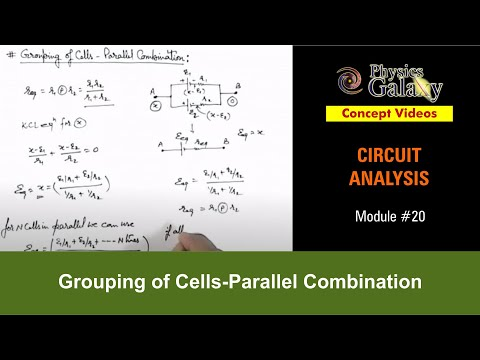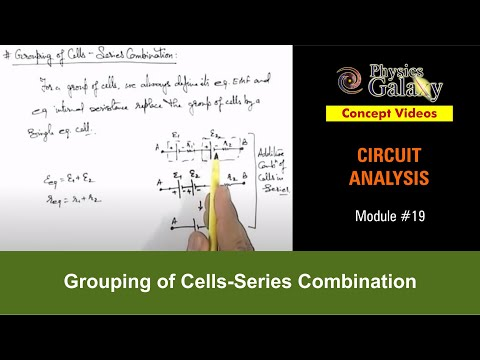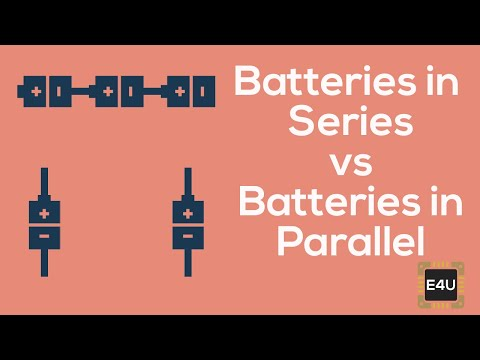## Combination of Cells

Subject: Science

#### Overview

The cells which are connected in such a way that the potential difference of the circuit is same as the cells used in the circuit but the time of current flowing increases is known as parallel combination. This note summarizes parallel and series combination of the cells.
##### Combination of Cells

To increase the amount of current or potential difference required in a circuit we can add cells to the circuit. This can be achieved in two different ways:

1. Parallel Combination:In parallel combination, the cells are connected in such a way that the potential difference of the circuit is same as the cells used in the circuit but the time of current flowing increases. The positive terminal of all the cells are connected to a single point and then connected to the positive terminal of the circuit and similarly, the negative terminal of all the cells are also connected to another single point and then connected to the negative terminal of the circuit. The cells that are used must have same potential difference. Cells with the different potential difference cannot be used in parallel combination. In this type of combination the potential difference between any two points in a circuit is the same and is equal to the individual potential difference of the cells used i.e. V = V1 = V2 = V3. In this combination the current provided by the cells is small and continuous for longer period of time. If we increase the number of cells the current cannot be increased but time of supply is increased.

2. Series combination:In series combination, the positive terminal of the first cell is connected to the negative terminal of the second cell and positive terminal of the second cell is connected to the negative terminal of the third cell and so on. In this combination of a cell, the overall potential difference is the sum of all the individual potential differences of the cells used in the circuit.
i.e. V = V1 + V2+ V3+ ...+ Vn.

3. . The current in the resistance in the series combination of cells is number of times the current due to individual cell. So, the current is larger but for shorter period of time. When we increase the number of cells in the series combination potential difference and current both increases.
##### Things to remember
• To increase the amount of current or potential difference required in a circuit we can add cells to the circuit. This can be achieved in two different ways:
• The cells which are connected in such a way that the potential difference of the circuit is same as the cells used in the circuit but the time of current flowing increases is known as parallel combination. (V = V1 = V2 = V3….)
• In series combination positive terminal of first cell is connected to the negative terminal of the second cell and positive terminal of second cell is connected to the negative terminal of third cell and so on. (V = V1 + V2 + V3 + …. )
• It includes every relationship which established among the people.
• There can be more than one community in a society. Community smaller than society.
• It is a network of social relationships which cannot see or touched.
• common interests and common objectives are not necessary for society.
##### Videos for Combination of Cells##### Electricity and Magnetism Numerical Type 1##### Electricity and Magnetism Numerical Type 2##### Electricity and Magnetism Numerical Type 3##### Electricity and Magnetism Numerical Type 4##### Grouping of Cells in Parallel way##### Grouping of Cells-Series Combination##### Series and parallel combination of battery cells
The combination of cells in which the negative terminal of two or more than two cells are at one point and the positive terminals at another point is known as parallel combination of cells.

The characteristics of parallel combination of cells are as follows: -

1. The potential difference between any two common points is always constant.
Total potential difference, V = V1 = V2 = V3
2. The current is provided to the external resistance (R) for a longer time. (If the total internal resistance of cells is neglected)
3. The magnitude of the electric current cannot be increased by a parallel combination of the cells.
The combination of cells in which the negative terminal of a first cell is connected with the positive terminal of second cell and the negative terminal of a second cell is connected to the positive terminal of a third cell and so on is known as series combination of cells.

Any three characteristics of series combination of cells are as follows: -

1. The total potential difference (voltage) is equal to the sum of the individual potential difference of the cells.

Total voltage (V) = V1 + V2 + V3

1. The current in the external resistance (R) is the number of times the current due to a single cell. Hence, this type of combination is used when current is required for a short time.
2. The magnitude of the  electric current can be increased by a series connection of cells.
Cells should be combined in series to increase the electromotive force (emf).
Following are three differences between parallel combination and series combination of cells are as follows: -
 Parallel combination of cells Series combination of cells In this combination, the positive terminals of the cells are connected to the one point and negative terminals to another point. In this combination, the negative terminal of one cell is connected to the positive terminal of another cell and so on. Current flowing through the circuit remains constant even when more cells are added. V = V1 = V2 = V3 Adding more cells can increase current flowing through the circuit. V = V1 + V2 + V3 It is used when current is required for long time. It is used when large amount of current is required.
The cells are connected in series combination in order to get maximum voltage.
Total voltage (V) = V1 + V2 + V3 + ……….Vn

Given,

;Potential difference (v) = 6V value of one resistance R1 = 3Ω

Value of second resistance R2 = 6Ω

(a) Total resistance R= R1 +R2

=3 + 6

= 9Ω

(b) Now from Ohm's law, we get V = IR

or, I= $\frac{V}{R}$

=$\frac{6}{9}$

= 0.67A

Hence, total resistance = 9Ω and current flowing through the circuit is 0.67 A